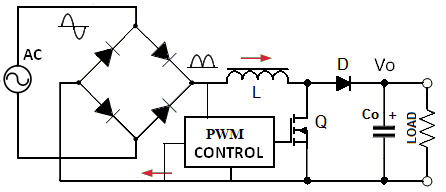# Power Factor Correction equipment (PFC)Power factor correction (PFC) can be done by passive or active circuits. Advances in semiconductor technology and low prices of control integrated circuits have made the use of active solutions for PFCs more appropriate for various applications.

### What is Power Factor (PF)?

The power factor (PF) is defined as the ratio between the real power and the apparent power in an electrical system. \

Since in AC circuits, the instantaneous current and the voltage vary continuously, the active power is calculated as the average value of the power as a function of time during a complete cycle of AC. The apparent power is simply the product of the RMS value of the current and the RMS value of the circuit voltage. It is a combination of active and reactive power. Reactive power is a function of the reactance of a circuit.

### Power Factor Correction:

The low power factor (PF) causes power losses in the distribution system. Some procedures can maintain the PF of an industrial electrical system at an acceptable level, such as:

• Minimize operation of lightly loaded motors,
• Operate equipment in their rated voltage and
• Install capacitors to decrease reactive power in AC circuits.

Some specific types of circuits can also be used to increase the PF of a system. The silicon controlled rectifier (SCR) or TRIAC are used in phase-controlled power supplies to control the phase of the input signal, which is filtered by an LC type filter.

One of the most commonly used PF correction circuits in current power supplies consists of a switched-mode step-up converter placed between the input rectifier and the storage capacitor.

An integrated circuit (IC) controls the converter so that the input current matches the waveform of the input voltage. A typical switched mode power supply (SMPS) without PF correction has a PF of around 0.6. The low PF together with the harmonics reduces the energy that can be provided to the loads.

### Boost Converter:

The use of a booster preconverter with a voltage higher than the input voltage can help avoid harmonic emissions. Boost converters are used for the correction of PF in discontinuous and continuous modes. When used in a discontinuous mode, the transistor (MOSFET) turns on when the inductor current reaches zero and turns off when it meets a certain reference.

This causes the input current to follow the waveform of the input voltage and the PF to approach 1.

On the other hand, when the power level of a switched-mode power supply is greater than 300W, an inverter Reinforcement that works in continuous mode is typically used. In this case, the current in the energy transfer inductor never reaches zero.

Scroll to Top